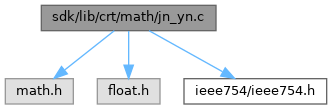ReactOS  0.4.15-dev-1201-gb2cf5a4
jn_yn.c File Reference
`#include <math.h>`
`#include <float.h>`
`#include "ieee754/ieee754.h"`
Include dependency graph for jn_yn.c:Go to the source code of this file.

## Typedefs

typedef int fpclass_t

## Functions

fpclass_t _fpclass (double __d)

int_errno (void)

double _jn (int n, double num)

double _yn (int order, double num)

## ◆ fpclass_t

 typedef int fpclass_t

Definition at line 5 of file jn_yn.c.

## ◆ _errno()

 int* _errno ( void )

Definition at line 19 of file errno.c.

20 {
22 }
Definition: tls.c:31

Referenced by _get_errno(), _set_errno(), and _yn().

## ◆ _fpclass()

 fpclass_t _fpclass ( double __d )

Definition at line 17 of file fpclass.c.

18 {
19  union
20  {
21  double* __d;
22  double_s* d;
23  } d;
24  d.__d = &__d;
25
26
27  /* With 0x7ff, it can only be infinity or NaN */
28  if (d.d->exponent == 0x7ff)
29  {
30  if (d.d->mantissah == 0 && d.d->mantissal == 0)
31  {
32  return (d.d->sign == 0) ? _FPCLASS_PINF : _FPCLASS_NINF;
33  }
34  /* Windows will never return Signaling NaN */
35  else
36  {
37  return _FPCLASS_QNAN;
38  }
39  }
40
41  /* With 0, it can only be zero or denormalized number */
42  if (d.d->exponent == 0)
43  {
44  if (d.d->mantissah == 0 && d.d->mantissal == 0)
45  {
46  return (d.d->sign == 0) ? _FPCLASS_PZ : _FPCLASS_NZ;
47  }
48  else
49  {
50  return (d.d->sign == 0) ? _FPCLASS_PD : _FPCLASS_ND;
51  }
52  }
53  /* Only remain normalized numbers */
54  else
55  {
56  return (d.d->sign == 0) ? _FPCLASS_PN : _FPCLASS_NN;
57  }
58 }
#define _FPCLASS_PN
Definition: float.h:76
#define _FPCLASS_NZ
Definition: float.h:73
#define _FPCLASS_PINF
Definition: float.h:77
#define _FPCLASS_NN
Definition: float.h:71
Definition: ieee.h:10
#define _FPCLASS_PZ
Definition: float.h:74
#define _FPCLASS_PD
Definition: float.h:75
#define d
Definition: ke_i.h:81
#define _FPCLASS_ND
Definition: float.h:72
#define _FPCLASS_NINF
Definition: float.h:70
#define _FPCLASS_QNAN
Definition: float.h:69

Referenced by _yn().

## ◆ _jn()

 double _jn ( int n, double num )

Definition at line 12 of file jn_yn.c.

13 {
14  /* FIXME: errno handling */
15  return __ieee754_jn(n, num);
16 }
GLdouble n
Definition: glext.h:7729
GLuint GLuint num
Definition: glext.h:9618
double __ieee754_jn(int n, double x)
Definition: jn_yn.c:64

## ◆ _yn()

 double _yn ( int order, double num )

Definition at line 21 of file jn_yn.c.

22 {
23  double retval;
24  if (!_finite(num)) *_errno() = EDOM;
25  retval = __ieee754_yn(order,num);
26  if (_fpclass(retval) == _FPCLASS_NINF)
27  {
28  *_errno() = EDOM;
29  retval = sqrt(-1);
30  }
31  return retval;
32 }
_STLP_DECLSPEC complex< float > _STLP_CALL sqrt(const complex< float > &)
Definition: complex.cpp:188
GLuint GLdouble GLdouble GLint GLint order
Definition: glext.h:11194
double __ieee754_yn(int n, double x)
Definition: jn_yn.c:227
#define EDOM
Definition: errno.h:39
_Check_return_ __MINGW_NOTHROW _CRTIMP int __cdecl _finite(_In_ double)
GLuint GLuint num
Definition: glext.h:9618
fpclass_t _fpclass(double __d)
Definition: fpclass.c:17
#define _FPCLASS_NINF
Definition: float.h:70
int * _errno(void)
Definition: errno.c:19